# 遗传算法与分枝定界法求解TSP研究Research on Solving TSP with Genetic Algorithm or Branch and Bound Method

DOI: 10.12677/CSA.2020.109169, PDF, HTML, XML, 下载: 98  浏览: 285

Abstract: When solving the traveling salesman problem, there are two commonly used methods, namely genetic algorithm method, and branch and bound method. To solve the given traveling salesman problem, this paper uses K-means clustering to improve the branch and bound method. By comparing the performance of these two algorithms in solving the TSP problem, the branch and bound method with K-means clustering performs better in solving the traveling salesman problem.

1. 引言

2. 问题描述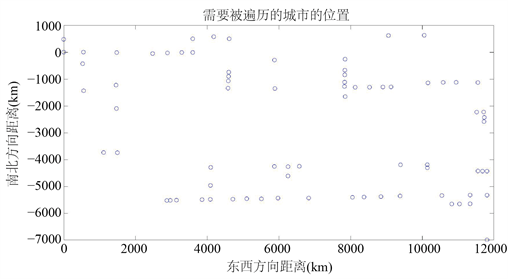Figure 1. The distribution of cities in TSP

3. 分支定界法

1. 从n个数据对象任意选择K个对象作为初始聚类中心。

2. 计算每个对象与聚类中心的距离；并根据最小距离重新对相应对象进行划分。

3. 重新计算每个聚类的均值作为新的聚类中心。

4. 循环2到3直到每个聚类不再发生变化为止。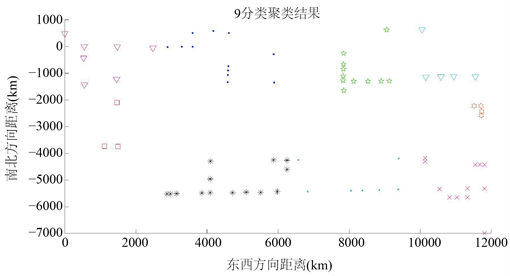Figure 2. The result of the first classification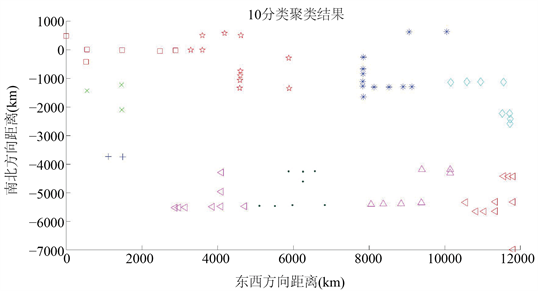Figure 3. The result of the second classification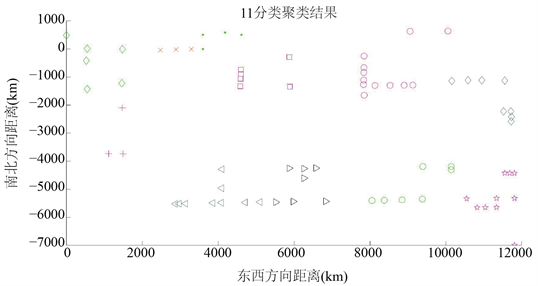Figure 4. The result of the third classification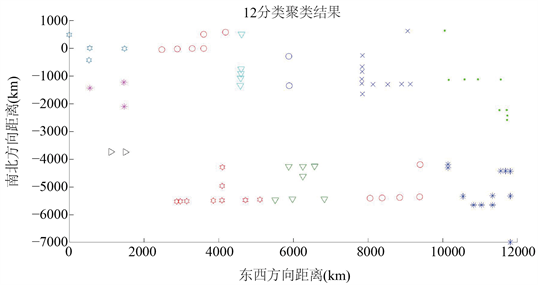Figure 5. The result of the forth classification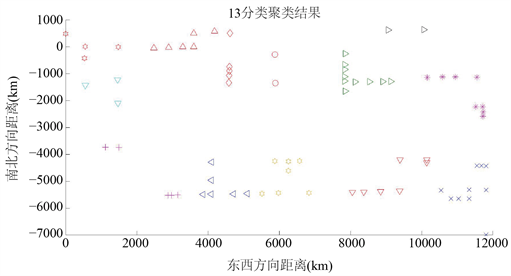Figure 6. The result of the fifth classification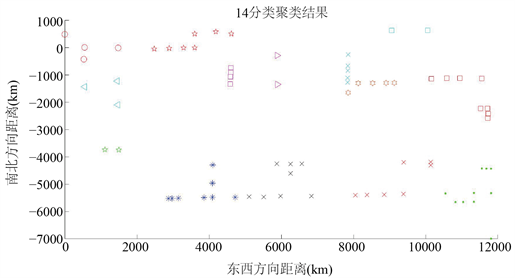Figure 7. The result of the sixth classificationFigure 8. Schematic diagram of branch and bound method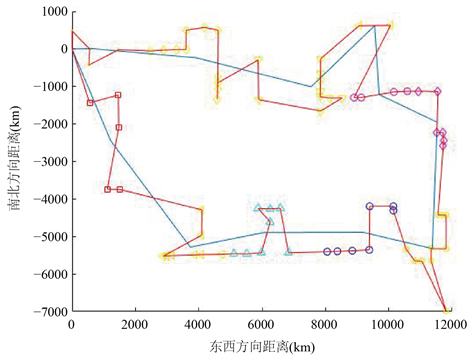Figure 9. The final path when the number of cluster categories is 11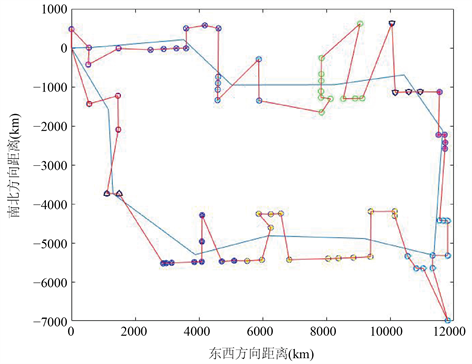Figure 10. The final path when the number of cluster categories is 12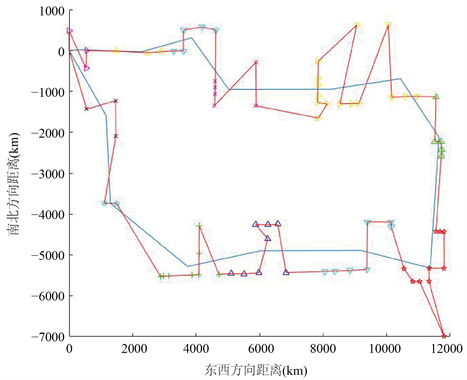Figure 11. The final path when the number of cluster categories is 13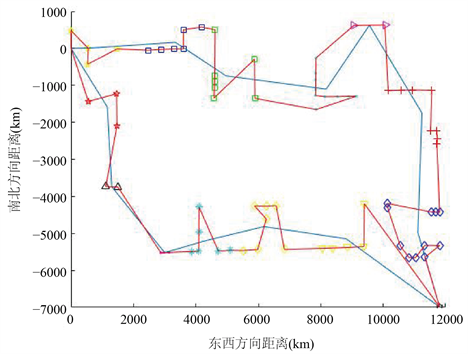Figure 12. The final path when the number of cluster categories is 14

4. 遗传算法

1. 将点的遍历顺序作为编码，即编码直接表示路径。

2. 根据每一编码计算其总路程，并用100000/log(总路程 + 1)作为适应度函数。

3. 在两个基因中随机选取两个交叉点，然后根据交叉点选取两个子串直接插入到对方的交叉点中。最后删掉两个后代中各自因交叉产生的重复的点。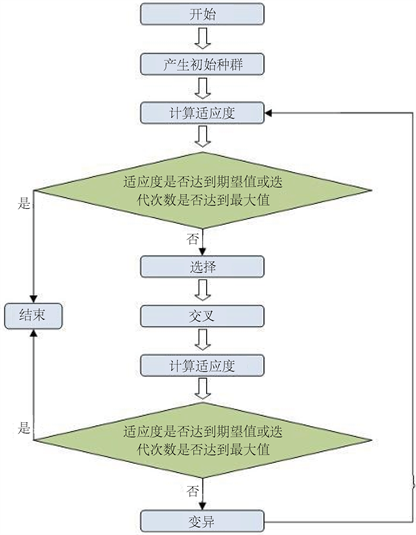Figure 13. The flowchart of genetic algorithm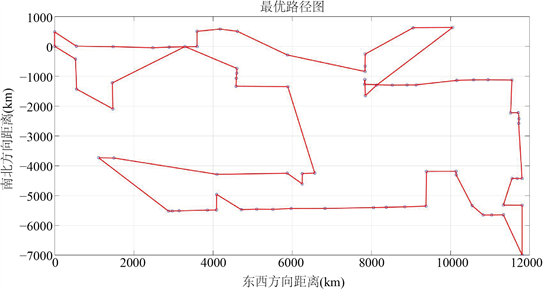Figure 14. The outcome of genetic algorithm

5. 结论

  许冲, 钟玮, 刘欣欣. 一种求解旅行商问题的演化算法研究[J]. 闽南师范大学学报(自然科学版), 2020, 33(2): 44-48.  姚宇威, 邓燕妮. 混合杂草遗传算法求解旅行商问题[J]. 科学技术创新, 2020(11): 40-41.  汤雅连, 杨期江. 求解旅行商问题的蚁群优化算法参数设计[J]. 东莞理工学院学报, 2020, 27(3): 48-54.  陈春燕, 彭阳, 许环梓, 何宇佳, 石苗. 蚁群和遗传算法在旅行路线规划中的研究[J]. 高师理科学刊, 2020, 30(7): 33-36  王兆锐, 林剑, 张俊丽, 官静萍. 基于车公里成本的多车型车辆规划方法[J]. 物流技术, 2019(1): 82-87.  胡士娟, 鲁海燕, 黄洋, 等. 求解工作量平衡多旅行商问题的改进遗传算法[J]. 计算机工程与应用, 2019(17): 150-155.